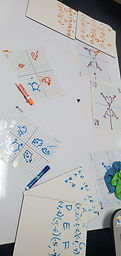## Mr. Jake

### Target 1​

###### Lesson Type:

New

Geometry

:

Symmetry

Graph symmetrical transformations on the coordinate plane.

###### 1:

Reflect a given figure across either the x- or y-axis and write the coordinates of the transformed image.

6th

###### Vocabulary:

Axis, reflection, coordinates

Activities:

Reflected shapes across the x-axis and y-axis

Determined what patterns exist when reflecting shapes on the coordinate plane### Home Exploration

###### Guiding Questions:## Absent Students:

Aaron

### Target 2

:

###### 1:

Utilize the standard algorithm to multiply decimal numbers (lining up the digits on the right).

###### 2:

Recognize that the decimal points in a multiplication problem do not need to be lined up vertically (like they do in an addition and subtraction problem).

###### 3:

Explain the process for determining where to place the decimal point in the product of a decimal problem.

###### 4:

Define the role that place-value plays in decimal computation.

5th

###### Vocabulary:

Decimal, multiplication

Activities:

Solved multiplication problems that included decimal values### Home Exploration

###### Guiding Questions:### Target 3

:

###### Vocabulary:

Activities:### Home Exploration